# Ncert Class 6 Math Data Handling Exercise 9.1

Data Handling Class 6 Ex. 8.4

New Ncert Class 6, Chapter 9 Math Free Solution.

Exercise 9.1

Question 1 :- In Mathematics-test, the following-marks were obtained by 40 students. Arrange these marks in a table using the tally-marks.(a) Find how many students obtained marks equal to or more than 7.
(b) How many students obtained marks below 4?

Solution 1:-

From the given data, we have the following table.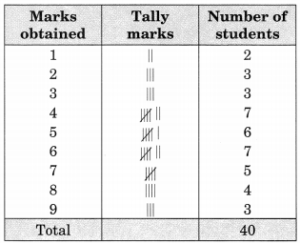(a) Number of students who obtained marks equal to or more than 7 = 5 + 4 + 3 = 12
(b) Number of students who obtained marks below 4 = 2 + 3 + 3 = 8.

Question 2 :- Following is the choice of sweets of 30-students, Class VI.

(a) Arrange the name of sweets in a table using tally-marks.
(b) Which sweet, preferred by most of the students?

Solution 2:-
(a) We have the following table :-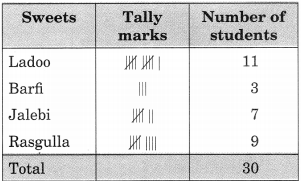(b) 11 students, or the majority of the students, like the Ladoo.

Question 3 :- Catherine threw, dice 40-times and noted the number appearing each  of the time as shown below: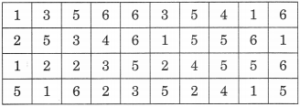Make a table and enter the data using tally-marks. Find the number, that appeared.
(а) The minimum number of times.
(b) The maximum number of times.
(c) Find-those-numbers; that appear an equal number of times.

Solution 3:-
We have the following table: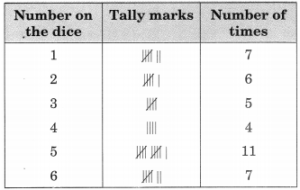From the above table, we get
(a) The minimum numbers of times = 4;
(b) The maximum numbers of times = 5;
(c) The number 1 and 6 appear for the same number of times, i.e., 7.

Question 4 :- Following pictograph; shows the number of tractors in five-villages :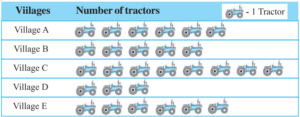Observe the pictograph and answer the following questions :
(a) Which village has the minimum-number of tractors?
(b) Which village has the maximum-number of tractors?
(c) How many more tractor village C, has as compared to village B?
(d) What is the total number of tractors in all the five-villages?

Solution 4:-
From the given pictograph, we have
(а) Village-D; has the minimum-number of tractors, = 3;
(б) Village-C; has the maximum number of tractors, = 8;
(c) Village-C; has 3-tractor more than that of the village-B;
(d) The total number of tractors in all the villages is = 28.

Question 5 :- The number of girl-students in each-class of a co-educational-middle-school is depicted by the pictograph:Observe this pictographs and answers the following questions:
(a) Which class has the minimum number of girl students?
(b) Is the number of girls in the Class-VI, less than the number of girls in Class-V?
(c) How many girls, are there in Class-VII?

Solution 5:-
(a) Class-VIII has the minimum-number of girl students = 1,(1/2) x 4 = 6;
(b) No, the number of girls in Class-VI; (4 x 4) = 16 and number of girls in Class-V; [2,(1/2) x 4] = 10
So, the number of girls student in Class-VI is not less than that of in Class-V.
(c) The number of girls, in Class-VII; (3 x 4) = 12.

Question 6 :- The sales of electric-bulbs, on different-days of a week is shown below: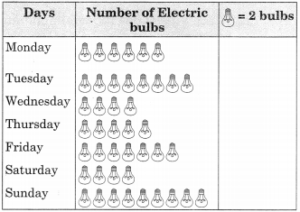Observe the pictograph; and answer the following-questions :
(a) How many bulbs were sold on Friday?
(b) On, which-day were the maximum-number of bulbs sold?
(c) On, which-day same number of bulbs were sold?
(d) On, which day minimum-number of bulbs were sold?
(e) If one big-carton can hold 9-bulbs. How many-cartons were needed in the given week?

Solution 6:-
(a) The number of bulbs sold on-Friday, (7 x 2) = 14;
(b) On-Sunday, the number of bulb sold, (9 x 2) = 18; which is maximum, in number;
(c) On, Wednesday and Saturday, the same-number of bulbs were sold, (4 x 2) = 8;
(d) The minimum-number of bulb were sold on Wednesday and Saturday, which is (4 x 2) = 8;
(e) Total number of bulbs-sold in a-week = 43;
Number of cartons needed = (43 x 2) ÷ 9 = 86 ÷ 9 = 9.56 = 10 cartons.

Question 7 :- In a village, six, fruit-merchants sold the following number of fruit-baskets in a particular season: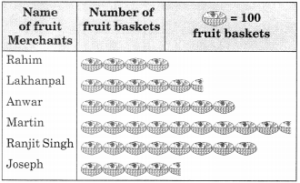Observe this pictographs, and answer the following questions :
(a) Which merchant sold the maximum-number of baskets?
(b) How-many fruit-baskets were sold by Anwar?
(c) The merchants who have sold 600 or more number of baskets are planning to buy a godown for the next-season. Can you name-them?

Solution 7 :-
(a) Martin sold the maximum-number of fruit-baskets, 9,(1/2) x 100 = 950;
(b) Number of fruit-baskets, sold by Anwar, (7 x 100) = 700;
(c) Anwar, Martin, and Ranjit Singh aim to purchase a godown after selling 600 or more fruit baskets.